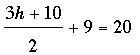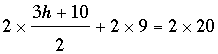SEARCH HOMEMath Central Quandaries & QueriesQuestion from CJ, a student: what is the solution for the equation 3h+10 divided by 2 +9=20CJ,I would like to eliminate the fraction so multiply each side by 2. This givesWhich becomes

3h + 10 + 18 = 40

Solve for h.

PennyMath Central is supported by the University of Regina and The Pacific Institute for the Mathematical Sciences.